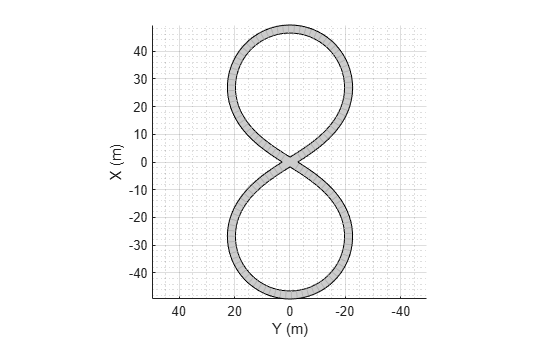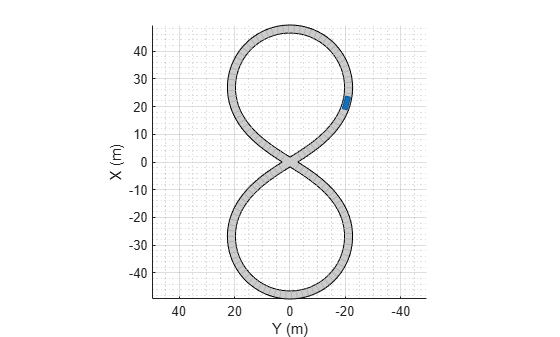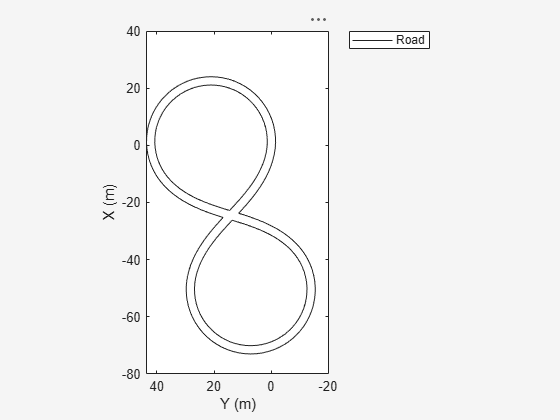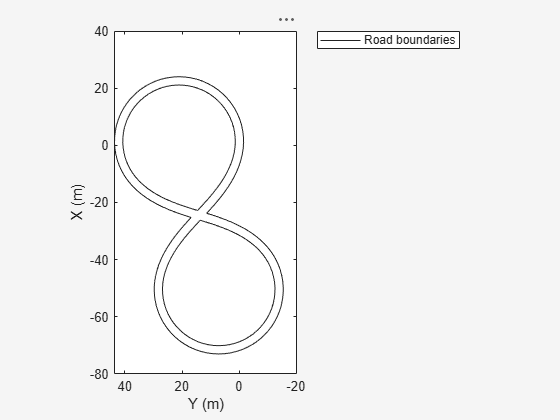Main Content

# driving.scenario.roadBoundariesToEgo

Convert road boundaries to ego vehicle coordinates

## Syntax

``egoRoadBoundaries = driving.scenario.roadBoundariesToEgo(scenarioRoadBoundaries,ego)``
``egoRoadBoundaries = driving.scenario.roadBoundariesToEgo(scenarioRoadBoundaries,egoPose)``

## Description

````egoRoadBoundaries = driving.scenario.roadBoundariesToEgo(scenarioRoadBoundaries,ego)` converts road boundaries from the world coordinates of a driving scenario to the coordinate system of the ego vehicle, `ego`.```

example

````egoRoadBoundaries = driving.scenario.roadBoundariesToEgo(scenarioRoadBoundaries,egoPose)` converts road boundaries from world coordinates to vehicle coordinates using the pose of the ego vehicle, `egoPose`.```

## Examples

collapse all

Create a driving scenario containing a figure-8 road specified in the world coordinates of the scenario. Convert the world coordinates of the scenario to the coordinate system of the ego vehicle.

Create an empty driving scenario.

`scenario = drivingScenario;`

Add a figure-8 road to the scenario. Display the scenario.

```roadCenters = [0 0 1 20 -20 1 20 20 1 -20 -20 1 -20 20 1 0 0 1]; roadWidth = 3; bankAngle = [0 15 15 -15 -15 0]; road(scenario,roadCenters,roadWidth,bankAngle); plot(scenario)```Add an ego vehicle to the scenario. Position the vehicle at world coordinates (20, –20) and orient it at a –15 degree yaw angle.

`ego = actor(scenario,'ClassID',1,'Position',[20 -20 0],'Yaw',-15);`Obtain the road boundaries in ego vehicle coordinates by using the `roadBoundaries` function. Specify the ego vehicle as the input argument.

`rbEgo1 = roadBoundaries(ego);`

Display the result on a bird's-eye plot.

```bep = birdsEyePlot; lbp = laneBoundaryPlotter(bep,'DisplayName','Road'); plotLaneBoundary(lbp,rbEgo1)```Obtain the road boundaries in world coordinates by using the `roadBoundaries` function. Specify the scenario as the input argument.

`rbScenario = roadBoundaries(scenario);`

Obtain the road boundaries in ego vehicle coordinates by using the `driving.scenario.roadBoundariesToEgo` function.

`rbEgo2 = driving.scenario.roadBoundariesToEgo(rbScenario,ego);`

Display the road boundaries on a bird's-eye plot.

```bep = birdsEyePlot; lbp = laneBoundaryPlotter(bep,'DisplayName','Road boundaries'); plotLaneBoundary(lbp,{rbEgo2})```## Input Arguments

collapse all

Road boundaries of the scenario in world coordinates, specified as a 1-by-N cell array. N is the number of road boundaries within the scenario. Each cell corresponds to a road and contains the (x, y, z) coordinates of the road boundaries in a real-valued P-by-3 matrix. P is the number of boundaries and varies from cell to cell. Units are in meters.

Ego vehicle, specified as an `Actor` or `Vehicle` object. To create these objects, use the `actor` and `vehicle` functions, respectively.

Ego actor pose in the world coordinates of a driving scenario, specified as a structure.

The ego actor pose structure must contain at least these fields.

FieldDescription
`ActorID`

Scenario-defined actor identifier, specified as a positive integer.

`Position`

Position of actor, specified as a real-valued vector of the form [x y z]. Units are in meters.

`Velocity`

Velocity (v) of actor in the x- y-, and z-directions, specified as a real-valued vector of the form [vx vy vz]. Units are in meters per second.

`Roll`

Roll angle of actor, specified as a real-valued scalar. Units are in degrees.

`Pitch`

Pitch angle of actor, specified as a real-valued scalar. Units are in degrees.

`Yaw`

Yaw angle of actor, specified as a real-valued scalar. Units are in degrees.

`AngularVelocity`

Angular velocity (ω) of actor in the x-, y-, and z-directions, specified as a real-valued vector of the form [ωx ωy ωz]. Units are in degrees per second.

For full definitions of these structure fields, see the `actor` and `vehicle` functions.

## Output Arguments

collapse all

Road boundaries in ego vehicle coordinates, returned as a real-valued Q-by-3 matrix. Q is the number of road boundary point coordinates of the form (x, y, z).

All road boundaries are contained in the same matrix, with a row of `NaN` values separating points in different road boundaries. For example, if the input has three road boundaries of length P1, P2, and P3, then Q = P1 + P2 + P3 + 2. Units are in meters.

Introduced in R2017a

## Support

#### Implementing an Adaptive Cruise Controller with Simulink

Download technical paper